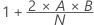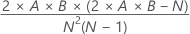# Methods and formulas for Runs Test

Select the method or formula of your choice.

## Observed number of runs

The observed number of runs is the number of groups of observations that are above or below the comparison criterion, K. Minitab uses the comparison criteria as a value you specify or the default mean of the observations. The number of runs in the sample helps determine whether the sample is random.

For example, a die is rolled 25 times. The scores are as follows:

• 4 6 3 2 2 1 6 4 4 5 1 1 4 5 3 1 5 6 6 3 4 4 5 2 3

Let the comparison criteria K = 3.5. The runs are as follows:

• (4 6) (3 2 2 1) (6 4 4 5) (1 1) (4 5) (3 1) (5 6 6) (3) (4 4 5) (2 3)

The first run (4 6) has values that are above K = 3.5, the second run (3 2 2 1) has values that are below K, and so on.

The observed number of runs = 10.

## Test statistics

### Formula

Minitab uses a normal approximation method to calculate the probability of getting an equal or greater number of runs. The probability is quite good if at least 10 observations exist both above and below K. The normal approximation for runs test is given by:

The p-value is the probability of observing the absolute value of a standard normal variable that is greater than the absolute value of Z.

If too few observations exist for the normal approximation to be valid, Minitab displays a message. Exact tables are widely available.

### Notation

TermDescription
ObservedThe number of runs in the sample
ExpectedVarianceAThe number of observations above K
BThe number of observations below or equal to K
NThe number of observations
By using this site you agree to the use of cookies for analytics and personalized content.  Read our policy